### Drawing Lots

January 9, 2023

Julius (Groucho) Marx (1890-1977) famously stated, "I don’t care to belong to any club that will have me as a member." Nobel Physics Laureate, Richard Feynman (1918-1988), had a different motive for his resignation from the US National Academy of Sciences. Feynman was by his nature against elitist organizations, and he resigned "because there was another organization most of whose time was spent in choosing who's illustrious enough to be allowed to join us..."

Long before paper ballots and hanging chads, voting was accomplished in ancient Greece through the use of readily available pebbles. Greeks were a seafaring people, as Homer's Iliad and Odyssey document, so there must have been an abundance of polished stones along the sea coast. Votes were cast by actually casting a pebble into an urn, a white pebble signifying a yes vote, and a black pebble signifying a no vote."Yea," or "nay." Polished white and black quartz pebbles.

Pebbles polished by flowing water, as in rivers and along coastlines, are not polished by the water, itself, but by sediments in the water. minerals of a certain Mohs hardness will be polished by minerals with the same or greater hardness.

(Portions of a Wikimedia Commons image by Mauro Cateb.)

Starting in the 19th century, some social organizations used a variant of this same pebble process. In a technological advance over pebbles, white and black marbles were used, and majority voting for admission of a new member was replaced by a process called blackballing. If just a single member objected, his solitary black marble was cause enough to reject an applicant.

This introduction brings us to the mathematics of drawing marbles at random from an urn containing an equal number of white and black marbles. In such a process, what's the probability of obtaining a streak of drawing n black marbles before a white marble is drawn? It's easily seen that the probability of getting a black marble at the first draw is 1/2, two black marbles in two draws is 1/4, etc., giving a simple geometric series. To get a true geometric series, a marble must be replaced after it's drawn. Alternatively, having a large number of marbles in the urn will give a good approximation if the drawn marbles are not replaced. The computer simulation of this is quite simple, and I give a C language program here with results shown in the figure.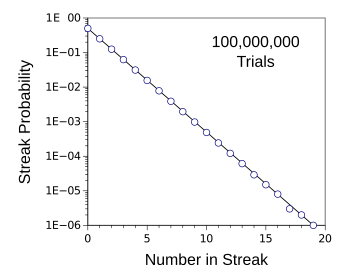Plot showing the probability of getting a streak of n black marbles pulled from an urn before a white marble is drawn.

This exponentially decaying function is best displayed on a semilog plot, as shown, since the probabilities descend quite rapidly.

These are data for a hundred million trials simulated with my C language program.

(Created using Gnumeric. Click for larger image.)

Semilog plots, such as the one above, are familiar to chemists, since they are used in the analysis of reaction rate constants. The Arrhenius rate equation, another exponential function, is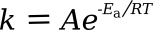in which k is the rate constant, A is a pre-exponential factor, Ea is the activation energy, R is the gas constant, and T is the absolute temperature. Plotting the natural logarithm of the rate constant against the reciprocal of the absolute temperature (1/T) gives a straight line whose slope is Ea/R and the intercept at 1/T = 0 is the natural log of A. Svante Arrhenius (1859-1927) was awarded the 1903 Nobel Prize for Chemistry for the theory of chemical equilibrium behind this simple equation.

The complement of exponential decay is exponential growth in which each successive term is proportionately greater. A common example of this is the wheat and chessboard problem in which one grain of wheat is placed on the first square, two on the second, four on the third, and so on. The total number of grains of wheat thus placed is 264 - 1, which is somewhat greater than 18 quintillion (US quintillion). This is sequence A000079 in the On-Line Encyclopedia of Integer Sequences.

While exponentially decaying function drop quite precipitously, there's another type of function with a large range on both plotted axes. This is the power law, given as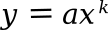in which a and k are both constants. Just as an exponential function is distinguished as a straight line in a semilog plot, a power law appears as a straight line in a log-log plot. Examples of a power law are the Stefan-Boltzmann law and the inverse-square laws of gravitational force and electrostatic force.

An interesting example of a power law is Zipf's law, named after American linguist, George Zipf (1902-1950). Zipf was the popularizer of the law, not its discoverer. Several wrote about it before him, including German physicist, Felix Auerbach (1856-1933). Zipf's law states that in any language the frequency of use of any word is inversely proportional to its rank in the list of word frequencies. In this power law, the probability of a word's occurrence is proportional to (1/n); thus, the most frequent word will occur about twice as often as the second most frequent word, three times as often as the third most frequent word, and so on. The power law exponent k for Zipf's law is -1.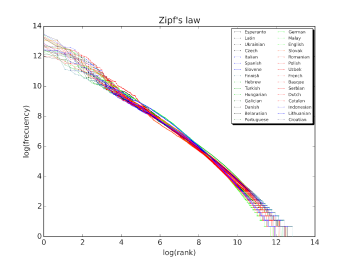Zipf's law for Wikipedia.

This is a plot of the rank versus frequency for the first 10 million words for 30 language versions of Wikipedia on October, 2015.

The straight line on this log-log plot shows power law behavior.

(Wikimedia Commons image by Sergio Jimenez. Click for larger image.)

Another example of power law behavior is company size, as indicated by the number of employees. This has an exponent of 1.06. The number of shares traded in a stock exchange per unit of time is a power law with and exponent of 1.5. The distribution of surnames in the United States, as shown in the following plot, also follows a power law.A plot of frequency vs rank for the first thousand surnames on the 2010 United States census showing power law behavior.

The ten names of highest rank, in rank order, are Smith, Johnson, Williams, Brown, Jones, Garcia, Miller, Davis, Rodriguez, and Martinez. The three Hispanic names are an indication of the shifting demographics of the United States.

(Created using Gnumeric. Also uploaded to Wikimedia Commons. Click for larger image.)

Sabin Roman of the Centre for the Study of Existential Risk of the University of Cambridge (Cambridge, United Kingdom) and the Odyssean Institute (London, United Kingdom), along with Francesco Bertolotti of the Carlo Cattaneo University (LIUC, Castellanza, Italy) have just published a paper on arXiv in which they present an algorithm for power law generation for drawing marbles from an urn. The trick is to add a large number of black marbles after every draw, thereby extending the tail of the distribution.

Their algorithm is as follows:

• Select a constant K. In the authors' example, K = 1.5. Then select the number of white W and black B marbles. In my demonstration program these are each 1000.

• The number of black marbles is increased by W/K after each draw, and the draws continue until a white marble is drawn. Black marbles are replaced after they are drawn.

• When a white marble is drawn, W and B are reset to their original values.

The computer simulation of this is as simple as that for drawing marbles at random from an urn, as presented earlier. I give a C language program here with results shown in the figure.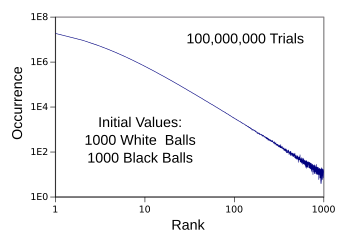Computer simulation results for the power law algorithm of ref. 3.

These are data for a hundred million trials simulated with my C language program.

(Created using Gnumeric. Click for larger image.)

### References:

Linked Keywords: Julius (Groucho) Marx (1890-1977); I don’t care to belong to any club that will have me as a member; Nobel Physics Laureate; Richard Feynman (1918-1988); motivation; motive; US National Academy of Sciences; elite; elitist; professional association; paper ballot; hanging chad; voting; ancient Greece; pebble; Greeks; seamanship; seafaring; population; people; Homer; Iliad; Odyssey; polishing; polished; rock (geology); stone; sea coast; cast; casting; urn; quartz; fluid dynamics; flow; flowing; water; river; coast; coastline; sediment; mineral; Mohs scale of mineral hardness; Mohs hardness; Wikimedia Commons; Mauro Cateb; 19th century; social; technology; technological; marble (toy); majority rule; majority voting; blackballing; mathematics; randomness; random; probability; winning streak; geometric series; approximation; computer simulation; C language program; drawing lots.c; plot (graphics); probability; exponential decay; exponentially decaying; function (mathematics); semilog plot; data; Gnumeric; chemist; analysis; chemical reaction; reaction rate constant; Arrhenius rate equation; rate constant; pre-exponential factor; activation energy; gas constant; thermodynamic temperature; absolute temperature; natural logarithm; multiplicative inverse; reciprocal; line (geometry); straight line; slope; y-intercept; Svante Arrhenius (1859-1927); award; awarded; Nobel Prize for Chemistry; scientific theory; chemical equilibrium<; equation; complement; exponential growth; proportionality (mathematics); proportionately; wheat and chessboard problem; food grain; wheat; square (geometry); names of large numbers; quintillion; A000079; On-Line Encyclopedia of Integer Sequences; precipitously; interval (mathematics); range<; Cartesian coordinate system; axis; axes; power law; constant (mathematics); log-log plot; Stefan-Boltzmann law; inverse-square law; gravitation; gravitational force; Coulomb's law; electrostatic force; Zipf's law; America; American; linguistics; linguist; George Zipf (1902-1950); popular science; popularizer; discovery (observation); discoverer; author; Germany; German; physicist; Felix Auerbach (1856-1933); language; statistical frequency; word; multiplicative inverse; inversely; ranking in statistics; rank; exponentiation; exponent; Wikipedia; plot (graphics); company; economic indicator; indicated<; employee; share (finance); stock trader; traded; stock exchange; unit of time; probability distribution; surname; United States; plot of frequency vs rank for the first thousand surnames on the 2010 United States census showing power law behavior; Hispanic; demographics of the United States; Gnumeric; Sabin Roman; Centre for the Study of Existential Risk; University of Cambridge (Cambridge, United Kingdom); Odyssean Institute (London, United Kingdom); Francesco Bertolotti; Carlo Cattaneo University (LIUC, Castellanza, Italy); scientific literature; publish; paper; arXiv; algorithm; methodology; trick; tail of the distribution; power law.c.